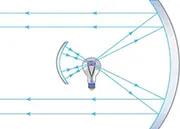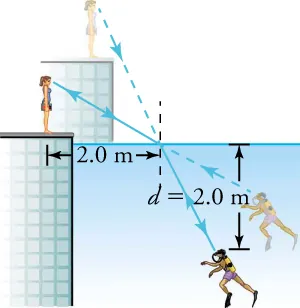Physics

# Extended Response

PhysicsExtended Response

### Extended Response

#### 16.1Reflection

43.

The diagram shows a lightbulb between two mirrors. One mirror produces a beam of light with parallel rays; the other keeps light from escaping without being put into the beam.Where is the light source in relation to the focal point or radius of curvature of each mirror? Explain your answer.

1. The bulb is at the center of curvature of the small mirror and at the focal point of the large mirror.
2. The bulb is at the focal point of the small mirror and at the focal point of the large mirror.
3. The bulb is at the center of curvature of the small mirror and at the center of curvature of the large mirror.
4. The bulb is at the focal point of the small mirror and at the center of curvature of the large mirror.
44 .
An object is placed $4.00\,\text{cm}$ in front of a mirror that has a magnification of $1.50$. What is the radius of curvature of the mirror?
1. $-24.0\,\text{cm}$
2. $- 4.80\,\text{cm}$
3. $4.80\,\text{cm}$
4. $24.0\,\text{cm}$

#### 16.2Refraction

45.

A scuba diver training in a pool looks at his instructor, as shown in this figure. The angle between the ray in the water and the normal to the water is 25°.What angle does the ray make from the instructor’s face with the normal to the water (n = 1.33) at the point where the ray enters? Assume n = 1.00 for air.

1. 68°
2. 25°
3. 19°
4. 34°
46.

Describe total internal reflection. Include a definition of the critical angle and how it is related to total internal reflection. Also, compare the indices of refraction of the interior material and the surrounding material.

1. When the interior material has a smaller index of refraction than the surrounding material, the incident ray may approach the boundary at an angle (called the critical angle) such that the refraction angle is 90° . The refracted ray cannot leave the interior, so it is reflected back inside and total internal reflection occurs.
2. When the interior material has a smaller index of refraction than the surrounding material, the incident ray may approach the boundary at an angle (called the critical angle) such that the refraction angle is less than 90° . The refracted ray cannot leave the interior, so it is reflected back inside and total internal reflection occurs.
3. When the interior material has the same index of refraction as the surrounding material, the incident ray approaches the boundary at an angle (called the critical angle) such that the refraction angle is less than 90° . The refracted ray cannot leave the interior, so it is reflected back inside and total internal reflection occurs.
4. When the interior material has a greater index of refraction than the surrounding material, the incident ray may approach the boundary at an angle (called the critical angle) such that the refraction angle is 90° . The refracted ray cannot leave the interior, so it is reflected back inside and total internal reflection occurs.

#### 16.3Lenses

47.

The muscles that change the shape of the lens in the eyes have become weak, causing vision problems for a person. In particular, the muscles cannot pull hard enough on the edges of the lens to make it less convex.

Part A—What condition does inability cause?

Part B—Where are images focused with respect to the retina?

Part C—Which type of lens corrects this person’s problem? Explain.

1. Part A—This condition causes hyperopia.
Part B—Images are focused between the lens and the retina.
Part C—A converging lens gathers the rays slightly so they focus onto the retina.
2. Part A—This condition causes myopia.
Part B—Images are focused between the lens and the retina.
Part C—A converging lens gathers the rays slightly so they focus onto the retina.
3. Part A—This condition causes hyperopia.
Part B—Images are focused between the lens and the retina.
Part C—A diverging lens spreads the rays slightly so they focus onto the retina.
4. Part A—This condition causes myopia.
Part B—Images are focused between the lens and the retina.
Part C—A diverging lens spreads the rays slightly so they focus onto the retina.
48 .
If the lens-to-retina distance is $2.00\,\text{cm}$, what is the power of the eye when viewing an object $50.0\,\text{cm}$ away?
1. $- 52.0\,\text{D}$
2. $0.52\,\text{D}$
3. $1.92\,\text{D}$
4. $52.0\,\text{D}$
Order a print copy

As an Amazon Associate we earn from qualifying purchases.